Next: Nomenclature: Up: Survey on Independent Component Previous: Conclusions

# Definition of Cumulants

In this appendix, we present the definitions of cumulants. Consider a scalar random variable of zero mean, say x, whose characteristic function is denoted by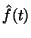: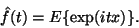(42)

Expanding the logarithm of the characteristic function as a Taylor series, one obtains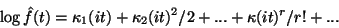(43)

where the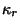are some constants. These constants are called the cumulants (of the distribution) of x. In particular, the first three cumulants (for zero-mean variables) have simple expressions: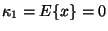(44)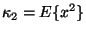(45)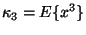(46)

Of particular interest for us is the fourth-order cumulant, called kurtosis, which can be expressed as [88,109]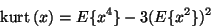(47)

Kurtosis can be considered a measure of the non-Gaussianity of x. For a Gaussian random variable, kurtosis is zero; it is typically positive for distributions with heavy tails and a peak at zero, and negative for flatter densities with lighter tails. Distributions of positive (resp. negative) kurtosis are thus called super-Gaussian (resp. sub-Gaussian).

Cumulants should be compared with (centered) moments. The r-th moment of x is defined as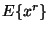[88,109]. (For simplicity, x was here assumed to have zero mean, in which case the centered moments, or moments about the mean, equal the non-centered moments, or moments about zero). The moments may also be obtained from a Taylor expansion that is otherwise identical to the one in (43), but no logarithm is taken on the left side. Note that the first 3 moments equal the first 3 cumulants. For r>3, however, this is no longer the case.

The mathematical simplicity of the cumulant-based approach in ICA is due to certain linearity properties of the cumulants . For kurtosis, these can be formulated as follows. If x1 and x2 are two independent random variables, it holds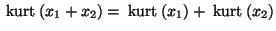and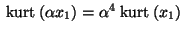, where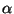is a scalar.

Cumulants of several random variables x1,...,xm are defined similarly. The cross-cumulant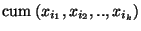for any set of indices ij is defined by the coefficient of the term ti1 ti2 ... tik in the Taylor expansion of the logarithm of the characteristic function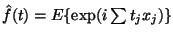of the vector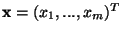.Next: Nomenclature: Up: Survey on Independent Component Previous: Conclusions
Aapo Hyvarinen
1999-04-23